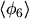## 2.3.3 Closure

Depending on the order up to which the moment equations are taken into account, transport models of different levels of sophistication are obtained. A characteristic of the moments method is that each equation for the momentof the distribution function contains the next higher moment. For example eqn. (2.104) contains the sixth order moment. Therefore the number of unknowns exceeds the number of equations and an expression for the highest occurring moment must be found. This can be achieved either by simplifying the equation of orderor by invoking some physical reasoning independent of the derivation of the moments themselves. This task is referred to as closure of the moment equations.

Subsections

M. Gritsch: Numerical Modeling of Silicon-on-Insulator MOSFETs PDF